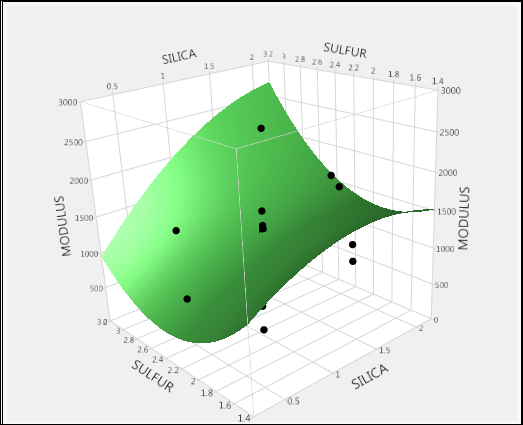Design of Experiments Guide > Response Surface Designs
Publication date: 07/30/2020

# Response Surface Designs

Response surface designs are useful for modeling quadratic surfaces. A response surface model can identify a point where a minimum or maximum value of the response occurs, if one exists inside the design region. Three distinct values for each factor are necessary to fit a quadratic function, so standard two-level designs are not appropriate for fitting curved surfaces.

Response surface designs are capable of fitting a second-order prediction equation for the response. The quadratic terms in these equations model the curvature in the true response function. If a maximum or minimum exists inside the design region, the point where that value occurs can be estimated.

Figure 11.1 Model for Response Surface Design Results